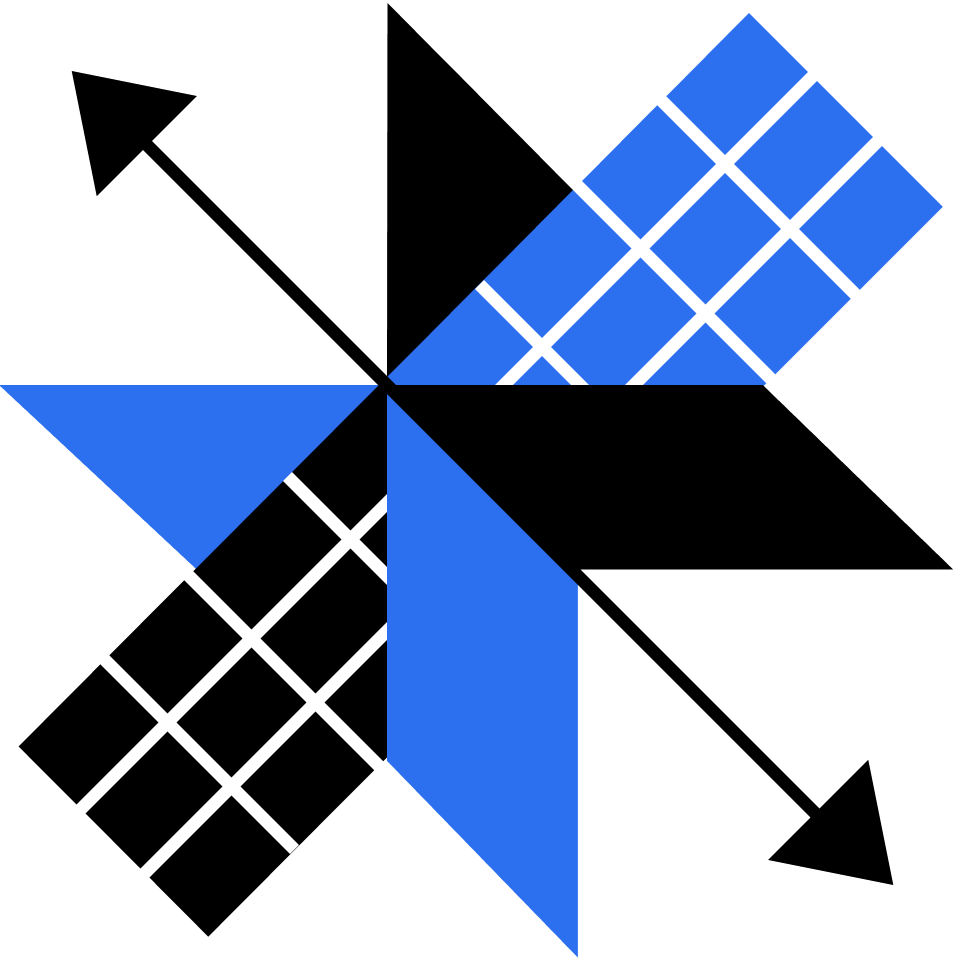## Reasoning with Algebra

Strengthen your algebra skills by exploring equations, exponents, and the unknown.

Balancing Scales

Elimination

Substitution

Graphing Rates

Equations of Lines

Special Lines

From Scales to Graphs

Number of Solutions

Problem Solving

Exponent Properties

Powers of Products and Powers

Zero and Negative Exponents

Problem Solving

Patterns

Describing Sequences

Arithmetic Sequences

Geometric Sequences

### Course description

Explore graphs of equations, exponents, counting problems, and more, emphasizing intuition and understanding over just finding an answer. This course will deepen your knowledge of basic algebra and introduce you to some surprisingly useful applications of this powerful mathematical tool. Some prior experience with algebra is assumed, but you're in good shape to start this course if you can plot points on a coordinate plane and use a variable to describe the relationship between the side length of a square and its area. By the end of this course, you’ll have extended your problem solving skills and be more aware of what common misconceptions can happen in algebra problems. You'll have enough background to go on to our Algebra II course.

### Topics covered

• Acceleration and Velocity
• Combinations
• Common Misconceptions
• Equations
• Exponents
• Factorials
• Lines
• Motion
• Permutations
• Scientific Notation
• Systems of Equations
• The Distributive Property

### Prerequisites and next steps

You should be comfortable with fundamental algebra concepts.

### Prerequisites

• Mathematical Fundamentals